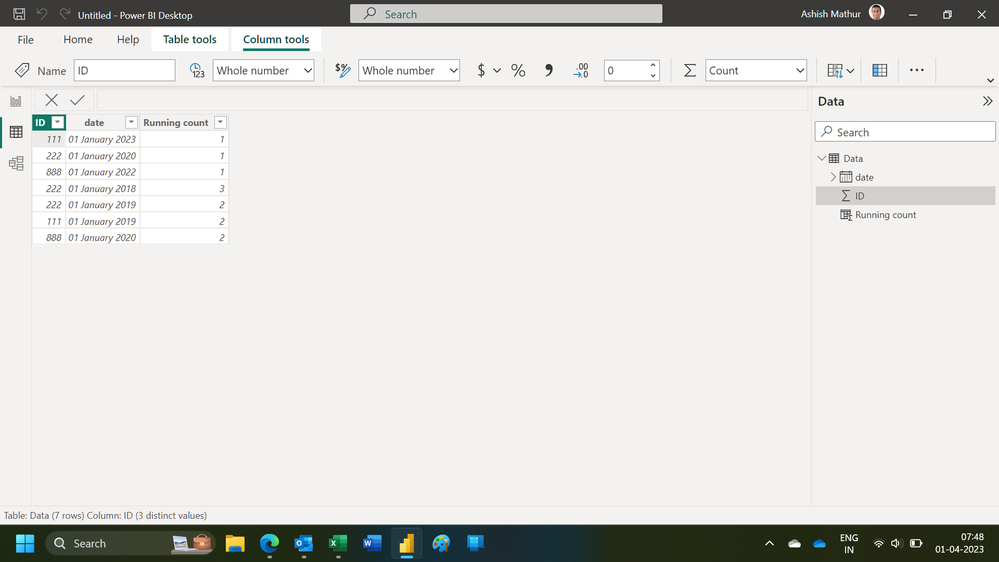cancel
Showing results for
Did you mean:Helper I

## Running count in Dax sort by date

Greetings,

How can I create a colmn in DAX for running count of IDs, sorted descending by the date. For example:

 ID date RUNNING COUNT 111 1/1/2023 1 222 1/1/2020 1 888 1/1/2022 1 222 1/1/2018 3 222 1/1/2019 2 111 1/1/2019 2 888 1/1/2020 2
1 ACCEPTED SOLUTIONSuper User

Hi,

Write this calculated column formula

``Running count = CALCULATE(COUNTROWS(Data),FILTER(Data,Data[ID]=EARLIER(Data[ID])&&Data[date]>=EARLIER(Data[date])))``

Hope this helps.Regards,
Ashish Mathur
http://www.ashishmathur.com
7 REPLIES 7Super User

Hi,

Write this calculated column formula

``Running count = CALCULATE(COUNTROWS(Data),FILTER(Data,Data[ID]=EARLIER(Data[ID])&&Data[date]>=EARLIER(Data[date])))``

Hope this helps.Regards,
Ashish Mathur
http://www.ashishmathur.comHelper I

@Ashish_Mathur The purpose of the running count is because I want to get the company name of the previous record for each employee. Would you please help me create the calculated column to get the previous company? below is an example:

 ID date RUNNING COUNT Company PREVIOUS COMPANY 111 1/1/2023 1 a b 222 1/1/2020 1 c a 888 1/1/2022 1 x y 222 1/1/2018 3 b 222 1/1/2019 2 a b 111 1/1/2019 2 b 888 1/1/2020 2 y

I tried this but it didn't work:

``````Previous company = CALCULATE(
maxx ('Table', 'Table'[Company]),
filter ( 'Table', 'Table'[ID] = EARLIER('Table'[ID])
&& 'Table'[Running Count] >= EARLIER('Table'[Running Count] )))``````Super User

Hi,

You do not need a running count column for that.  Try this calculated column formula

``Previous Company = LOOKUPVALUE(Data[Company],Data[date],CALCULATE(MAX(Data[date]),FILTER(Data,Data[ID]=EARLIER(Data[ID])&&Data[date]<EARLIER(Data[date]))),Data[ID],Data[ID])``

Hope this helps.Regards,
Ashish Mathur
http://www.ashishmathur.comHelper I

@Ashish_Mathur Thank you! This is exactly what I wanted.Super User

You are welcome.

Regards,
Ashish Mathur
http://www.ashishmathur.comSuper User

@Maha1 , a new measure

countx(filter(allselected(Table), Table[ID] = max(Table[ID])  && Table[Date] >= max(Table[Date]) ) , Table[ID])

You can also consider window function

Power BI Window function Rolling, Cumulative/Running Total, WTD, MTD, QTD, YTD, FYTD: https://youtu.be/nxc_IWl-tTcHelper I

thank you